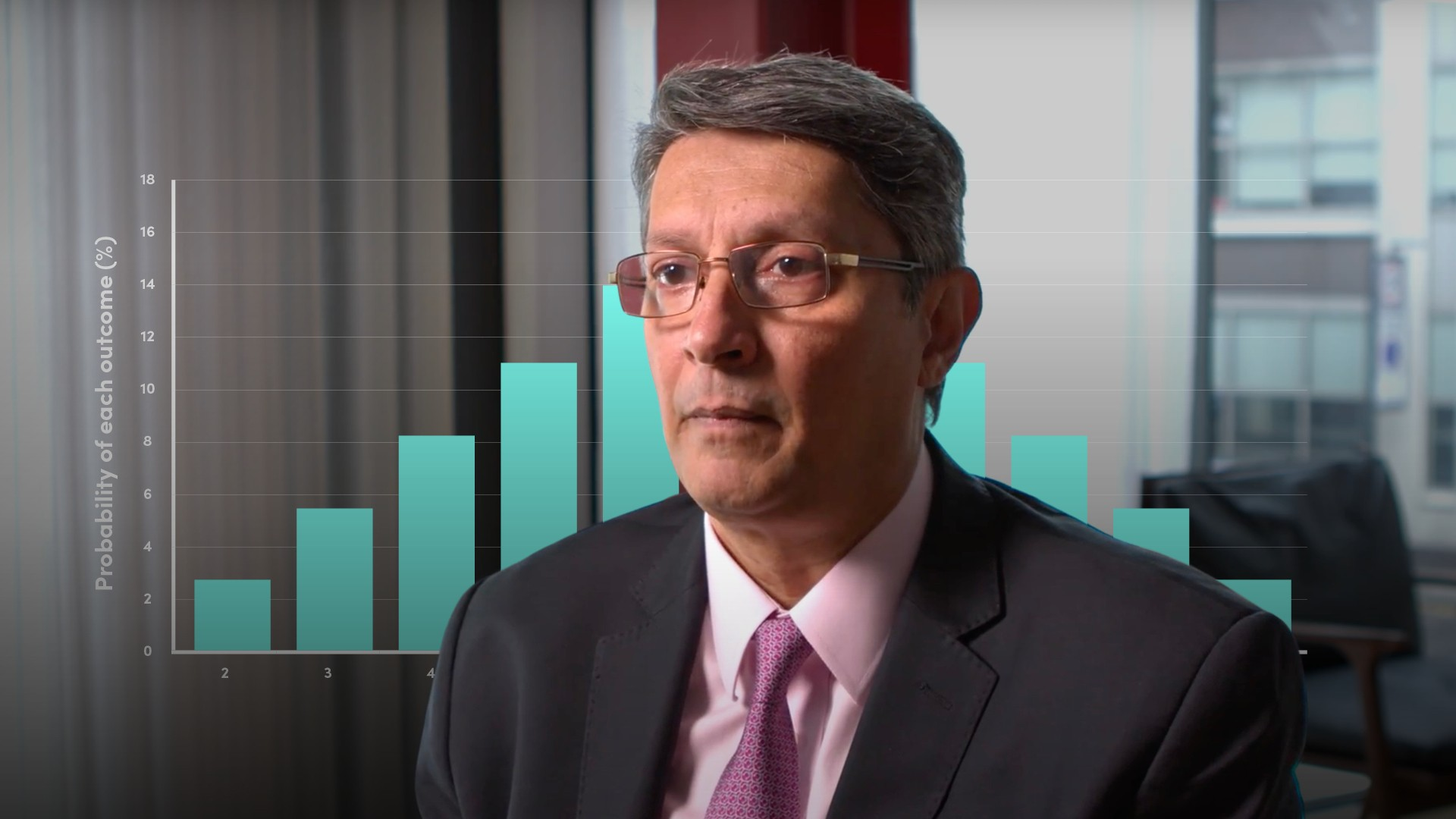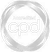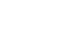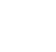# Cumulative Distribution Function### Abdulla Javeri

In the previous video of the series, Abdulla introduced the concept of probability distributions. In this video, Abdulla will expand and explore the cumulative distribution function. He explains how to calculate it and how to use it by building on the example featured in the video on the basics of probability.

Financial MathsIn the previous video of the series, Abdulla introduced the concept of probability distributions. In this video, Abdulla will expand and explore the cumulative distribution function. He explains how to calculate it and how to use it by building on the example featured in the video on the basics of probability.

Speak to an expert

Speak to an expert today to access this and all of the content on our platform.### Cumulative Distribution Function

4 mins 36 secs

Overview

The cumulative distribution function shows the area under the curve by adding all the individual probabilities in an experiment.

Key learning objectives:

• Explain the basics of cumulative distribution function

• Understand how it is visually represented

## Speak to an expert

Speak to an expert today to access this and all of the content on our platform.

Summary

#### What is the Cumulative Distribution Function?

The cumulative distribution function integrates the area under the curve. Integration in this respect is just a fancy way of saying, adding up the area under the curve, adding the individual probabilities. The cumulative distribution involves adding the probabilities of each outcome starting from the left-hand side of the x axis moving to the right. It gives the total area under the curve to the left of any given point that we choose. Therefore, the area to the right of that point will be 100% minus the area to the left. It climbs up and eventually tapers off at 100%.

#### What are the uses of the CDF?

It allows us to answer questions such as what’s the probability of an outcome being x, less than x or greater than y, or, somewhere in between x and y etc.

#### How do the probabilities differ for continuous variables?

For continuous variables, where these intervals are infinitely small, each individual probability will be very close to zero and the total area under the curve will be very close to 100%.

#### How is this visually represented?## Speak to an expert

Speak to an expert today to access this and all of the content on our platform.### Abdulla Javeri

Abdulla’s career in the financial markets started in 1990 when he entered the trading floor of the London International Financial Futures Exchange, LIFFE, and qualified as a pit trader in equity and equity index options. In 1996, Abdulla became a trainer for regulatory qualifications and then for non-exam courses, primarily covering all major financial products.

There are no available videos from "Abdulla Javeri"

### Accredited by